# Sohcahtoa Worksheet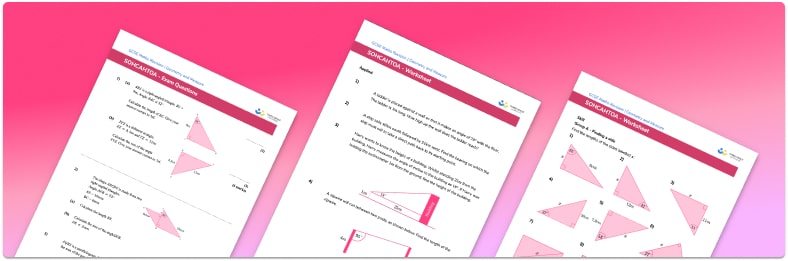Help your students prepare for their Maths GCSE with this free comparing SOHCAHTOA worksheet of 34 questions and answers

• Section 1 of the comparing SOHCAHTOA worksheet contains 27 skills-based SOHCAHTOA questions, in 3 groups to support differentiation
• Section 2 contains 4 applied SOHCAHTOA questions with a mix of worded problems and deeper problem solving questions
• Section 3 contains 3 foundation and higher level GCSE exam style SOHCAHTOA questions
• Answers and a mark scheme for all SOHCAHTOA questions
• Follows variation theory with plenty of opportunities for students to work independently at their own level
• All questions created by fully qualified expert secondary maths teachers
• Suitable for GCSE maths revision for AQA, OCR and Edexcel exam boards
• To receive this resource and regular emails with more free resources, blog posts and other Third Space updates, enter your email address and click below.

• This field is for validation purposes and should be left unchanged.

You can unsubscribe at any time (each email we send will contain an easy way to unsubscribe). To find out more about how we use your data, see our privacy policy.

### SOHCAHTOA at a glance

SOH CAH TOA is a mnemonic used to help us remember the different trigonometric ratios: sin(x)=opposite/hypotenuse, cos(x)=adjacent/hypotenuse and tan(x)=opposite/adjacent.

These trigonometric ratios can be used to calculate the size of missing sides and missing angles in right-angled triangles. The first step is to label the opposite side, the adjacent side and the hypotenuse for the given angle. Once we have labelled the sides, we can use SOHCAHTOA to decide which trigonometric function to use. This depends on which sides we know or are trying to work out.

When finding the length of a side of a right angled triangle, we substitute the information we know into the appropriate trig ratio and solve the equation to find the missing length.  When finding the size of an angle, again we substitute the information we know into the appropriate trig ratio and we then use the inverse trigonometric functions, sin-1, cos-1 or tan-1 to solve for the angle.

Trigonometry has many real life applications, for example finding side lengths and angles of elevation in construction.

Looking forward, students can then progress to additional geometry worksheets and practice problems for example a 3D shapes worksheet or an area of a triangle worksheet.For more teaching and learning support on Geometry our GCSE maths lessons provide step by step support for all GCSE maths concepts.

## Related worksheets

Exact Trig Values Worksheet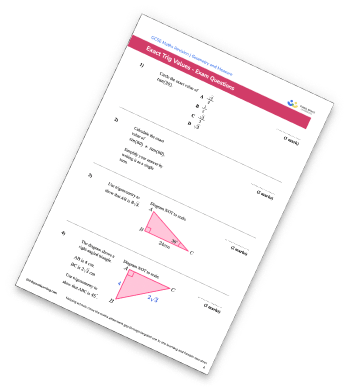Hypotenuse Worksheet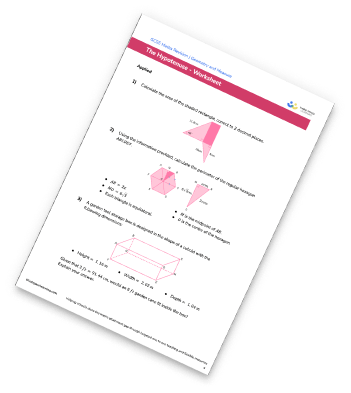Trigonometry Worksheet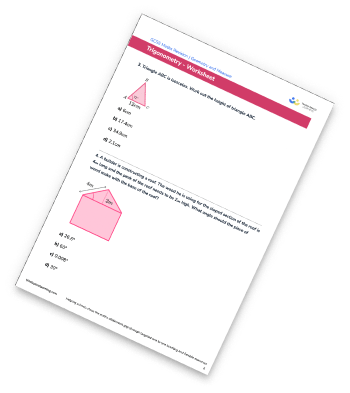Sine Rule Worksheet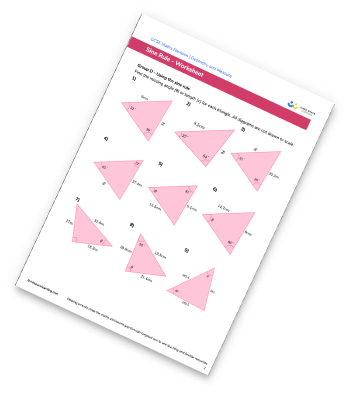## Do you have KS4 students who need more focused attention to succeed at GCSE?There will be students in your class who require individual attention to help them succeed in their maths GCSEs. In a class of 30, it’s not always easy to provide.

Help your students feel confident with exam-style questions and the strategies they’ll need to answer them correctly with our dedicated GCSE maths revision programme.

Lessons are selected to provide support where each student needs it most, and specially-trained GCSE maths tutors adapt the pitch and pace of each lesson. This ensures a personalised revision programme that raises grades and boosts confidence.

Find out more